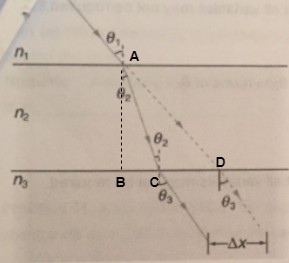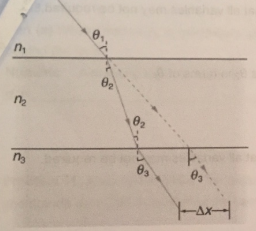# Problem:  Calculate the distance the ray is displaced, in millimeters by the glass, given that the incident angle is 40.0 the glass is 0.95 cm thick. Take 1.52 for the index of refraction for crown glass.

###### FREE Expert Solution

Snell's law:

$\overline{){{\mathbf{\eta }}}_{{\mathbf{1}}}{\mathbf{s}}{\mathbf{i}}{\mathbf{n}}{{\mathbf{\theta }}}_{{\mathbf{1}}}{\mathbf{=}}{{\mathbf{\eta }}}_{{\mathbf{2}}}{\mathbf{s}}{\mathbf{i}}{\mathbf{n}}{{\mathbf{\theta }}}_{{\mathbf{2}}}}$

For easy reference, let's label our figure as below:(1)sin(40.0°) = (1.52) sin (θ2)

θ2 = sin-1 [sin(40.0°)/1.52] = 25°

99% (157 ratings)###### Problem DetailsCalculate the distance the ray is displaced, in millimeters by the glass, given that the incident angle is 40.0 the glass is 0.95 cm thick. Take 1.52 for the index of refraction for crown glass.